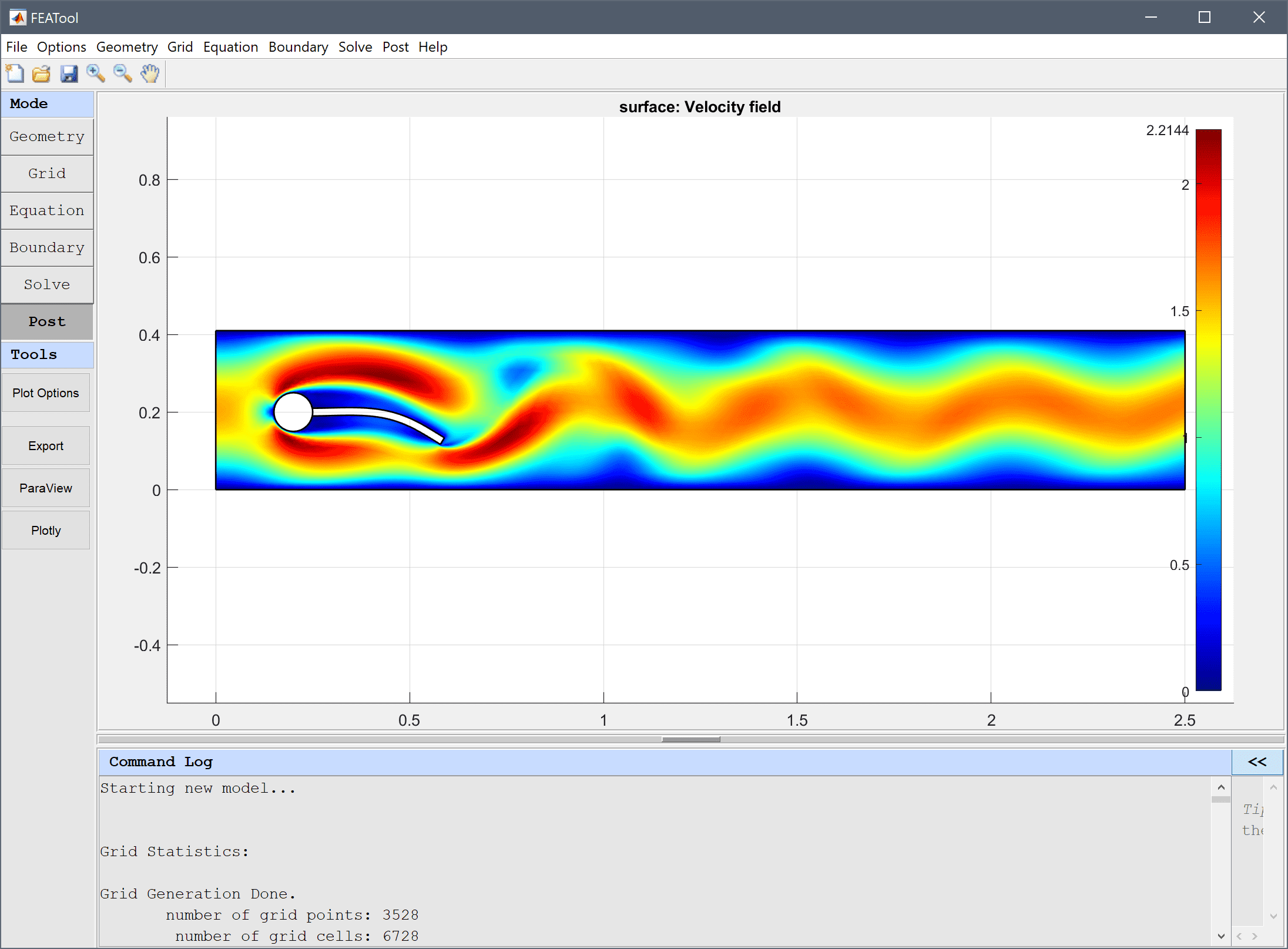# Fluid-Structure Interaction - Elastic Beam## Model Data

Physics Modes: fluid structure interaction

Keywords: nonlinear elasticity fluid structure

Fluid-structure interaction benchmark problem for stationary, laminar, and incompressible flow around a cylinder with an attached elastic beam. Although it is not possible to derive an analytical solution to these test cases, numerical solutions to benchmark reference quantities have been established for the beam deformation, drag, and lift forces .

The test configurations consider a solid cylinder centered at (0.2, 0.2) with diameter d = 0.1 in a l = 2.2 by h = 0.41 rectangular channel. The fluid is assumed to have a constant density and viscosity, and a fully developed parabolic velocity profile is prescribed at the inlet. A beam of length 0.4 and width 0.02 is fixed to the end of the cylinder. First a static solution with a Reynolds number Re = 20 is considered, and then an instationary solution with Re = 100.

This model is available as an automated tutorial by selecting Model Examples and Tutorials… > Multiphysics > Fluid-Structure Interaction - Elastic Beam from the File menu, and also as the MATLAB simulation m-script example ex_fluidstructure1. Step-by-step tutorial instructions to set up and run this model are linked below.## Reference

 Hron J. A monolithic FEM/multigrid solver for ALE formulation of fluid structure interaction with application in biomechanics. In H.-J. Bungartz and M. Schaefer, editors, Fluid-Structure Interaction: Modelling, Simulation, Optimisation, LLNCSE. Springer, 2006.